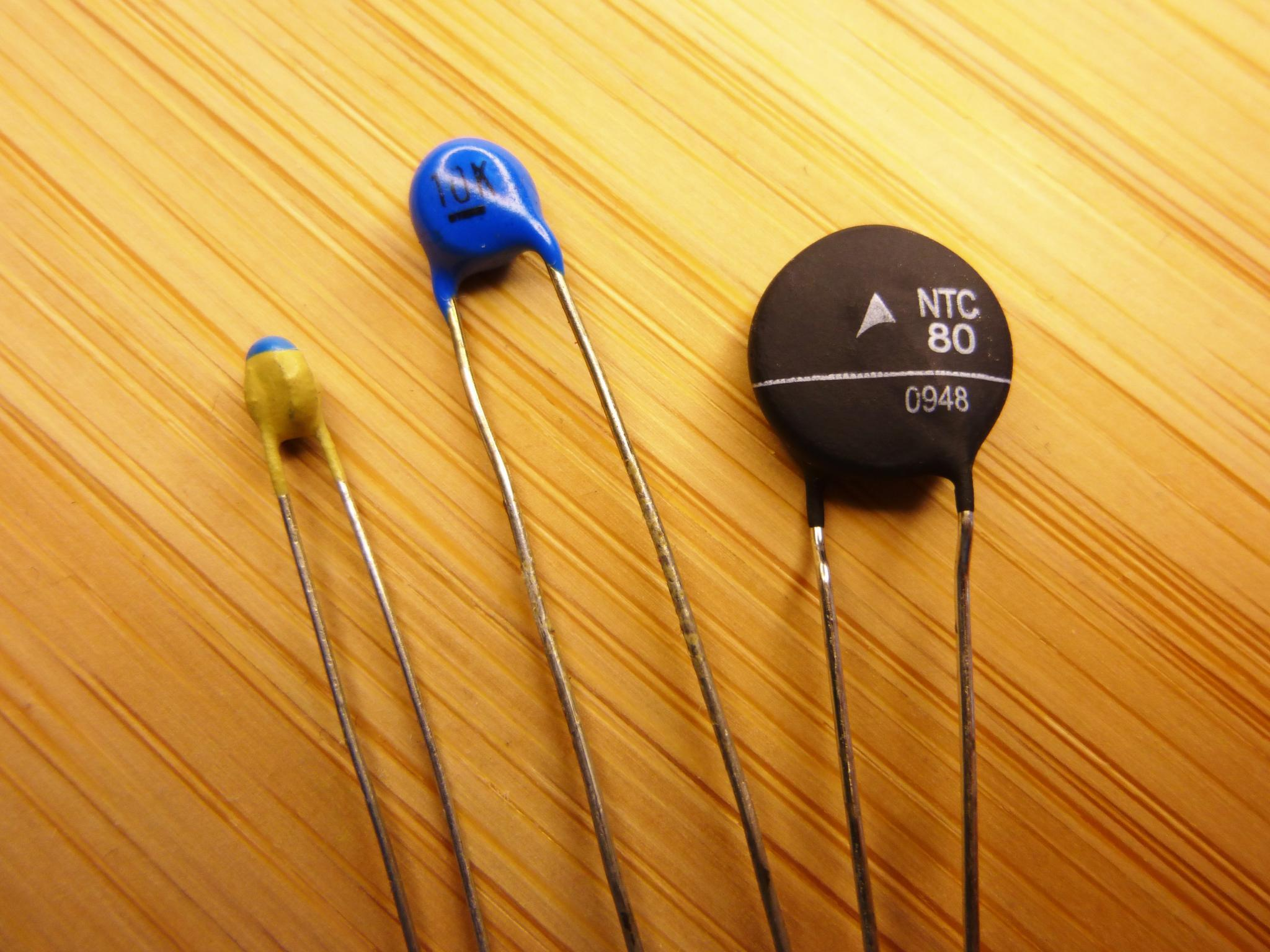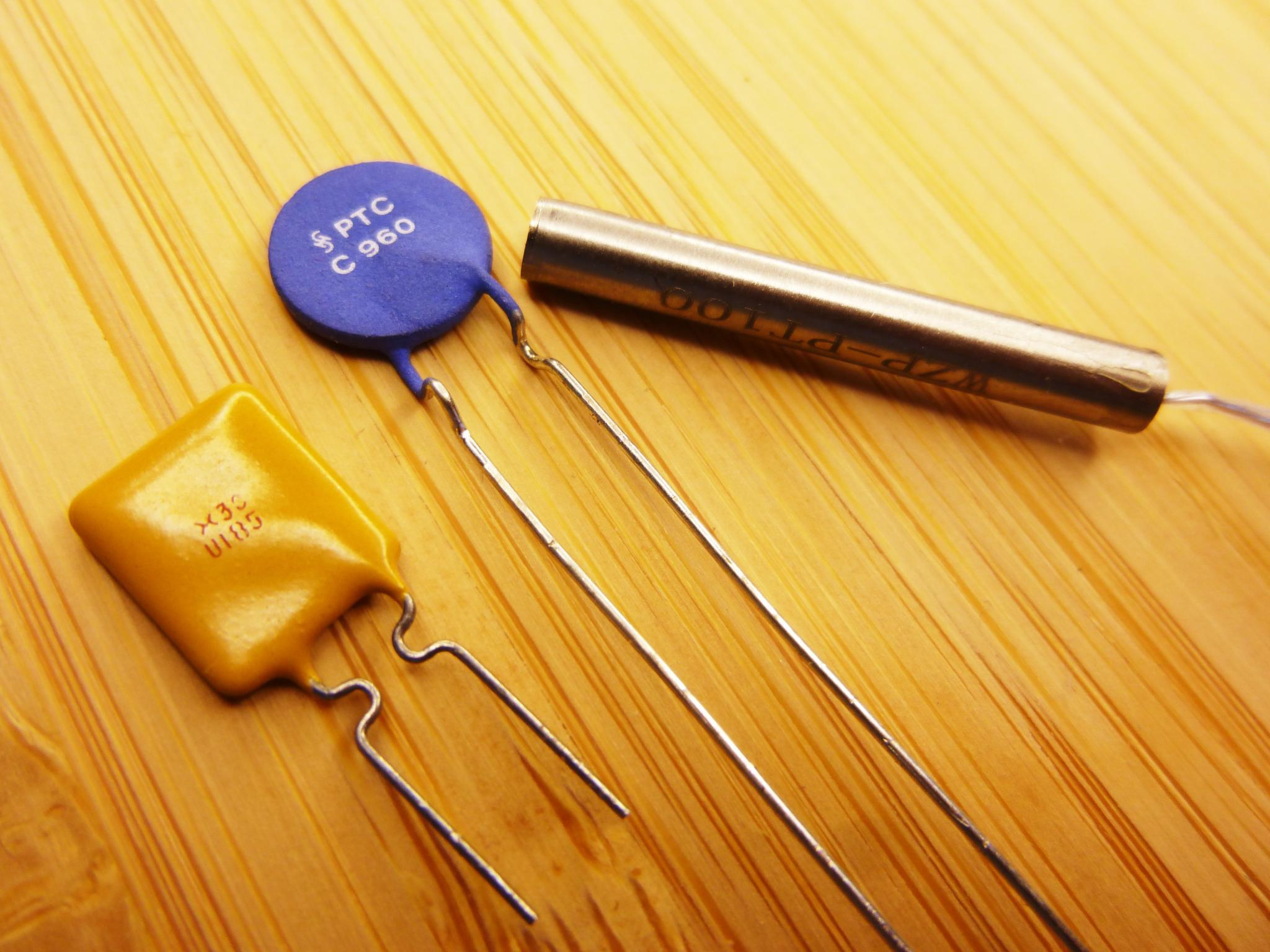# Thermistors

The resistivity of materials is dependent on temperature. Thermistors are electric components which make use of this.

## Thermistors

When we spoke about ohms law, I noted that not all electric components comply to it. Even resistors do not fully obey it and this is because there is another external factor that can change the resistance value: temperature. The resistance of normal resistors does not change a lot as they are usually constructed in a way that minimizes the temperature effect. There are, however, other electrical components which actively use the fact that the resistance of different materials changes with temperature. These components are called thermistors.In this tutorial we take a look at different types of thermistors and their usage. We won't look into the details of why they behave in the way they do, however. A lot of thermistors use semiconductor materials and the explanation of their temperature behavior can get a lengthy and complicated. Truth is, for using them we don't need that in depth knowledge about how they work. Let's keep it simple.

Thermistors can be into two categories: negative temperature coefficient thermistors (NTCs) and positive temperature coefficient thermistors (PTCs). The resistance of material compositions with a positive temperature coefficient increases with temperature. This behavior can be observed in conventional light bulb, for example. You can try it if you want. Just measure the current that flows through the bulb at a constant voltage. Turn on the light bulb and you can see that the current decreases as the bulb heats up. Conventional light bulb typically use a tungsten filament, but other metals share the property of a positive temperature coefficient. There are also materials that exhibit a negative temperature coefficient. Their resistance decreases with temperature. Pure silicon or germanium, but also carbon have this property. Most NTCs use electroceramics with semi conductor properties, like metal oxides.

## Negative Temperature Coefficient Thermistors (NTCs)

NTC thermistors are very common for temperature measurement applications. If you have an analog temperature sensor module for your Arduino, then it almost certainly uses an NTC thermistor. The circuit symbol used in schematics includes a little -t° which reminds us of the negative temperature coefficient of these thermistors.In the picture below you can see different NTCs. Let's have look at how they are commonly used.### Temperature Sensors

The two NTCs on the left of the picture are used for temperature sensing. The blue one has a resistance of 10 kΩ and the other one a resistance of 1 MΩ at 25 °C. To measure temperature, one can use a voltage divider circuit to determine the resistance. Note, that NTCs have a non-linear resistance curve. With higher temperature their resistance drops faster and faster. A temperature conversion table for the different resistance values is usually given in the datasheet. There is also a conversion formula that can be used to calculate the temperature in software. We will look into that in the next tutorial.

What's the different between these to NTCs and the third one? The third one has a resistance of only 80 Ω at 25 °C. If we wanted to use it in a voltage divider, a pretty high current would be flowing through the divider. This causes the NTC to heat up. In temperature measurement circuits this will cause incorrect temperature readings. For that reason NTCs for temperature measurement applications usually have a high resistance. But what is the purpose of this 80 Ω NTC?

### Inrush Current Limiting

Another possible application for NTCs is inrush current limiting and this is what the 80 Ω resistor is for. In circuits with big capacitors, inductors or motors you may experience high current when powering them up. This current spike may damage other components and can be prevented by using an NTC as inrush current limiter. The NTC gets connected in series with the load. On start the NTC is at room temperature and will have a resistance around 80 Ω. This reduces the maximum current that can flow through it on power up. After a short time, the NTC will heat up due to the current flowing through it and its resistance will decrease and finally become very small. The circuit can then operates with its full power.

## Positive Temperature Coefficient Thermistors (PTCs)

The second category of thermistors are PTCs. Their symbol is shown below and as you can see there is a small +t° to make clear that this is a thermistor with a positive temperature coefficient.Below you can see different PTC and only one of them is used for temperature measurement. There are a lot of interesting applications for PTCs, so let's talk about them first, before we talk about using PTCs as temperature sensor.### Current Limiting

PTCs can also be used for current limiting. Obviously not in the same way as NTCs are. PTCs behave different in that their resistance increases with a rising temperature. This means that their resistance will increase if a high current flows through them. You can place a PTC in series to a load to limit the maximum current. If the load causes a high current the PTCs resistance will increase and thus limit the current that can flow through the circuit.

An extreme example for current limiting are PPTCs (Polymer PTCs) that are used as self-resettable fuses. The very left PTC in the picture is such a self-resettable fuse. These PTC use a polymer material with carbon particles in it. At normal temperature their resistance is very small. When used in a circuit they will heat up if current flows through them. Up to a certain current the air takes the produced heat away and the PPTC resistance stays low. If the current increases, however, this is no longer possible and the PPTC heats up rapidly. It trips and its resistance increases to a very high value. There will be almost no current flowing through the PPTC anymore, until it cools down and becomes conductive again. Due to this behavior PPTCs are used as replacement for fuses in a lot of electric products. In case of an over current or short circuit event the PPTC will cut off the current flow. In difference to a normal fuse the PPTC allows the device to be usable again after some minutes. The downside is, that PPTC take a comparably long time to heat up before they trip. Traditional fuses usually react a lot faster.

Not all PTC have a resistance curve, where the resistance increase that rapidly. But the fact, that PTC are self-limiting, allows the use of them in circuits where traditionally a resistor is used for current limiting. When using a PTC instead we can be quite sure that it won't burn up like a resistor would in case of an overload situation. We do not always need a fuse like behavior. Normal PTCs like the one in the middle can do the job too. A common material for them is barium titanate an electroceramic. This material is also used in PTC heaters. These electrical heater have the advantage they won't overheat when driven with a constant voltage. The increasing resistance of the material and the resulting current limiting behavior, limits their maximum temperature.

### Temperature Sensors

Let's finally have look at PTC temperature sensors. A very common one in industrial applications is the Pt100 resistance temperature detector (RTD). This and similar sensors use different metals and metal alloys. With increasing temperature their resistance increases slightly. While they can be used for very accurate measurements and have an almost linear resistance curve, their main problem is that the change in resistance per °C is only very small. The Pt100 sensor use platinum for temperature sensing and has a resistance of 100 Ω at 0 °C. The resistance change, however, is only 0,385 Ω/°C. This requires a more precise measurement circuit than a simple voltage divider. NTCs are a cheaper and easier to use alternative, especially if you don't need the high accuracy of the Pt100.

A possible alternative to are silicon based PTCs like the KTY sensor series. Their resistance change per °C is higher than the one of classic RTDs which makes them easier to use. They are however very sensitive to electrostatic discharge (ESD). The resistance curve in the usable temperature range is a parable, but they are actually way more linear than NTCs. They sometimes use the TO-92 packaging and therefore look a bit like transistor. As only two connections are needed the middle pin is sometimes cut off. They then look like a transistor with only two legs. If you have seen one already, you might have wondered about that. The big advantage of these sensors is that they can be potentially integrated into other chips. With ceramic NTCs or PTCs this is not possible.